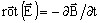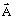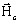# Transient Magnetic: solved equations (vector model 2D)

## Introduction

The vector model is the general model proposed for a 2D application.

## Equation solved with vector model (2D applications)

The Maxwell-Faraday equationimplies presence of electric scalar potential V, such as:The equation solved by the finite elements method in a Transient Magnetic application is written:where:

• ν0 is the tensor of the relative reluctivity of the medium
• ν0 is the reluctivity of the vacuum; ν0 = 1/μ0 = 1/(4 π 10-7) (in m/H)
•is the vector potential (in Wb/m)
•is the coercive magnetic field (permanent magnets) (in A/m)
• σ is the tensor of the conductivity of the medium (in S)
• V is the electric scalar potential (in V)

## State variables, vector model (2D)

The state variables are:

• the magnetic vector potential• the electric scalar potential V

The state variables, dependent on the problem type, plane 2D or axisymmetric 2D, are given in the table below.

Type of the problem State variable

Notation (in Flux 3D)

plane An AN1*
axisymmetric r⋅An RAN1*
Note: *Index 1 means that the quantity is a real quantity.